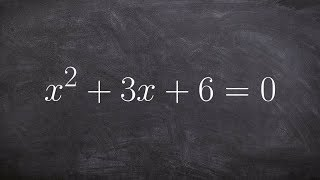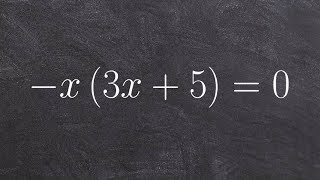# Related Videos

## Solve the quadratic equation $-x^2+7x-10=0$

Go!
Go!
1
2
3
4
5
6
7
8
9
0
a
b
c
d
f
g
m
n
u
v
w
x
y
z
.
(◻)
+
-
×
◻/◻
/
÷
2

e
π
ln
log
log
lim
d/dx
Dx
|◻|
θ
=
>
<
>=
<=
sin
cos
tan
cot
sec
csc

asin
acos
atan
acot
asec
acsc

sinh
cosh
tanh
coth
sech
csch

asinh
acosh
atanh
acoth
asech
acsch

### Videos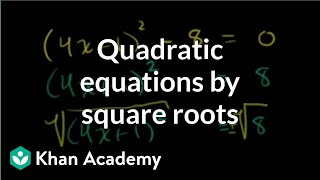### Solving quadratic equations by square roots | Algebra II | Khan Academy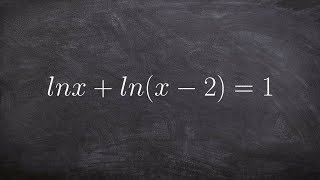### Solving a natural logarithmic equation using the quadratic formula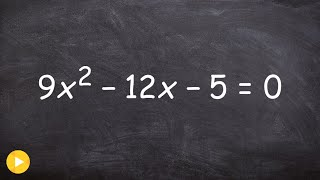### Algebra 2 - Learn the ac method for factoring and solving a quadratic equation 9x^2‐12x‐5=0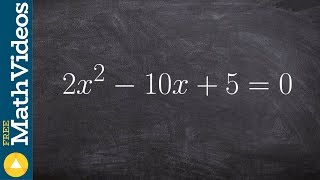### Algebra 2 - Learn how to complete the square by factoring out a term 2x^2-10x+5=0